Next: Limiting cases Up: Odd-even staggering of binding Previous: Odd-even staggering filters

# Theoretical models

In this section, we investigate several exactly solvable models to see the interplay between the particle-hole and particle-particle channels of interaction. In all cases, the Hamiltonian has the form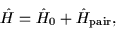(13)

where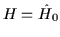is either the intrinsic (i.e., deformed) single-particle Hamiltonian or the laboratory-system quadrupole-quadrupole Hamiltonian, and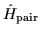is always the monopole-pairing (seniority) Hamiltonian: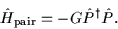(14)

In Eq. (14) G is the pairing strength parameter,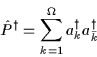(15)

denotes the monopole-pair creation operator, and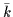denotes the time-reversed state.

Properties of the Hamiltonian (13) depend on the ratio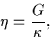(16)

where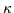represents the strength of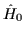. For both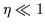(weak pairing) and(strong pairing), one can treat the Hamiltonian (13) perturbatively. However, the situation encountered most often in the nuclear physics context is the intermediate case (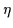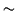0.4) in which pairing correlations are strongly influenced by the nuclear mean field.Next: Limiting cases Up: Odd-even staggering of binding Previous: Odd-even staggering filters
Jacek Dobaczewski
2000-03-09